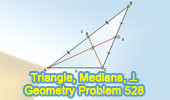## Wednesday, October 13, 2010

### Problem 528: Triangle, Medians, Perpendicular, Measurement

Geometry Problem
Click the figure below to see the complete problem 528 about Triangle, Medians, Perpendicular, Measurement.Complete Problem 528

Level: High School, SAT Prep, College geometry

#### 1 comment:

1.Solution sent by Peter Tran:

Let G is the intersection of AD and CE
We have AD^2= ½*(AB^2+AC^2-BC^2/2) …….. Formula to calculate the median